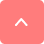# How to Copy Equations from PDF to Word without Formatting Issues

#### Summary :

You can’t directly copy mathematical equations from PDF to Microsoft Word, you need some tricks. On this page, learn from the step-by-step guide to do so without formatting issues.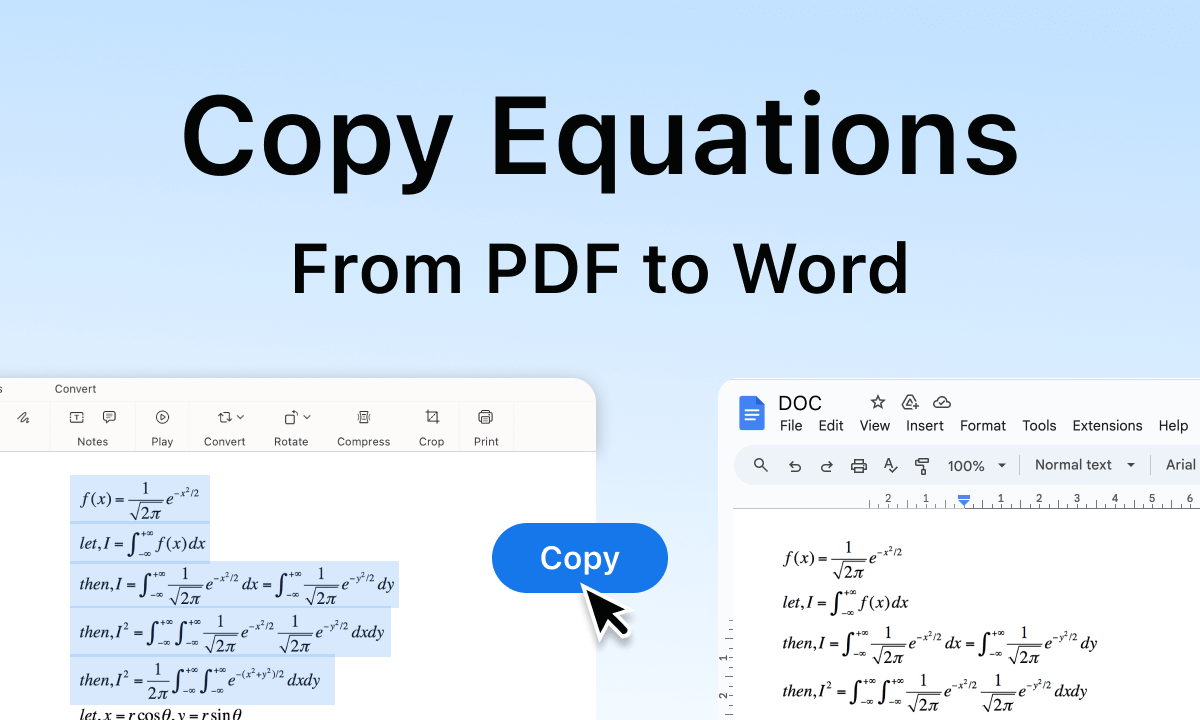How to Copy Equations from PDF to Word

Given the complex nature of mathematical notations, it’s difficult to accurately transfer an equation between the two document formats, and copying mathematical equations from a PDF file to a Word document can be a challenging task.

Luckily, this page will cover the specialized tools and techniques for you to do the trick. From the step-by-step tutorials, you’ll easily learn how to copy mathematics equations from PDF into Microsoft Word for proper editing and processing.

👉To save time, you can directly download the free PDF editor software used to transform PDF with equations into Microsoft Word without formatting issues.

## Copy Equations from PDF Using Mathpix

MathPix is a popular snipping program on Windows and Mac that is used to copy math and chemistry from your computer screen, it works by converting images to editable formats like LaTex, MS Word, HTML, and Markdown.

With Mathpix, you get to directly extract equations from images and PDFs, and no longer have to manually type or input each equation, saving you time and reducing the likelihood of errors.

There’s a free plan available at Mathpix, offering up to 10 Snips and up to 10 PDF pages.

Step 1. Download and install Mathpix Snip from the Mathpix official website, and then you’ll be asked to create an account.

Step 2. Open the PDF document you need in any PDF reader program, here I’ll use Microsoft Edge browser.

Step 3. Open the Mathpix Snipping Tool, and click on the upper-right snipping button.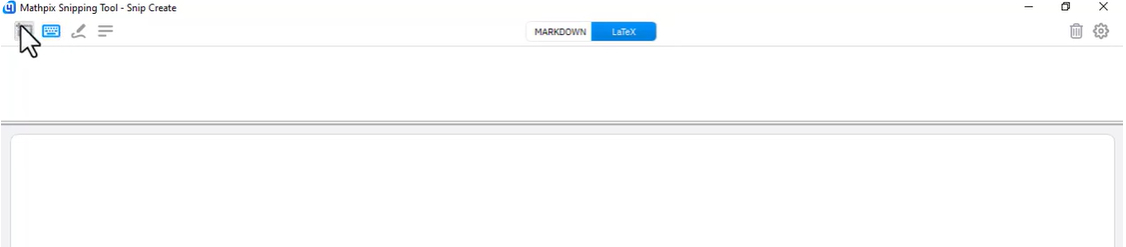Open Mathpix Snip Tool

Step 4. Click and hold the cursor to draw an area on your PDF to extract the math equation.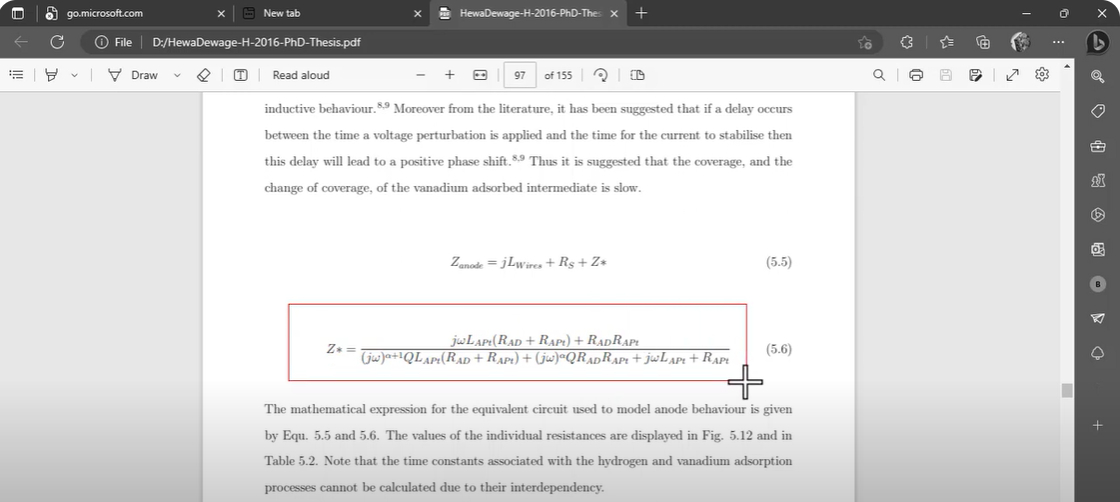Extract Equation from PDF Using Mathpix

Step 5. Voila! Now that Mathpix Snip has precisely extracted the PDF equation, click the lower right Copy MS Word to copy the text in Microsoft Word format. Alternatively, click the Export .docx button to export a Word document.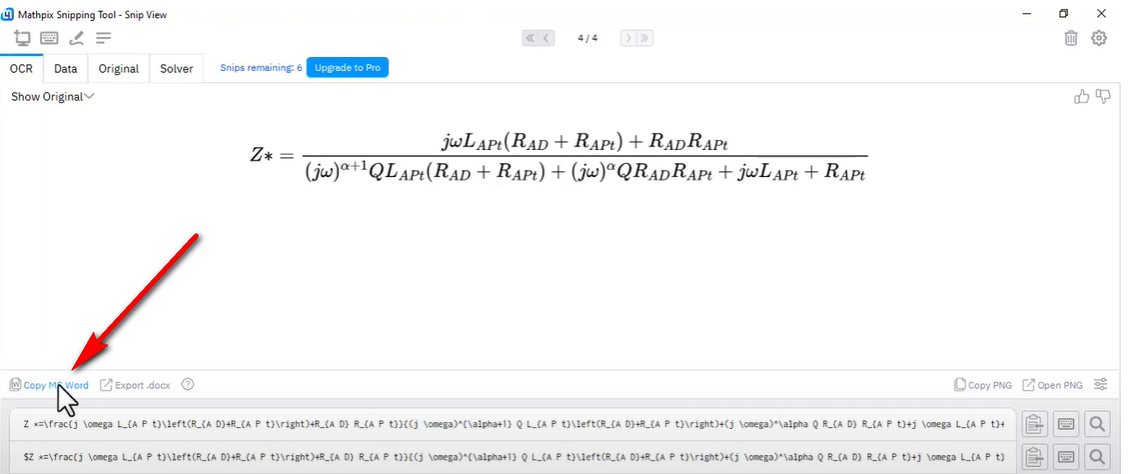Copy PDF Equation in Microsoft Word Format

Step 6. Now create a new Microsoft Word document, and use the keyboard shortcut Ctrl + V (Windows) or Command + V (Mac) to paste the copied text.

Step 7. That’s it! Now you’ll get a fully editable math equation in Microsoft Word.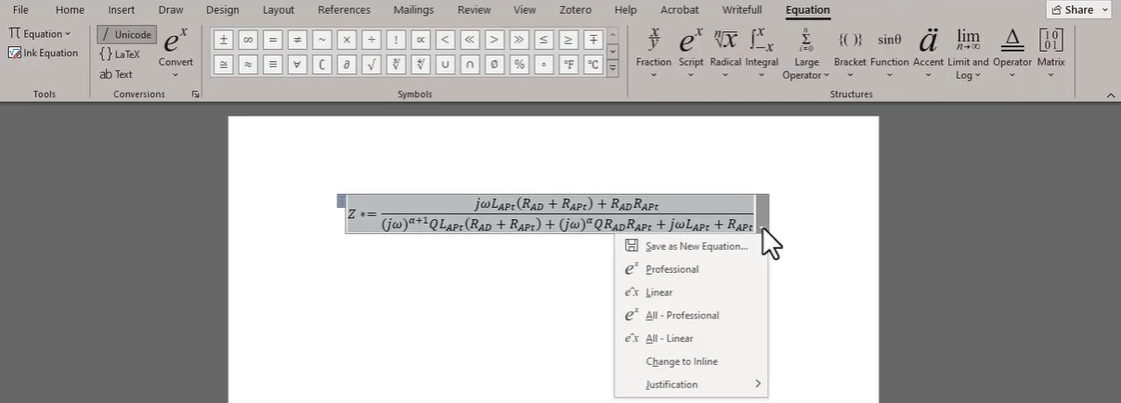Paste Equation from Mathpix to Word

## Convert PDF with Math Equations to Word

Another practical method is by converting the PDF to Word, with a tool that supports text with mathematical equations.

For this, you need the best free PDF converter software PDFgear Desktop, which allows you to convert math equations in PDFs to Microsoft Word documents with the original font and layouts, and without any formatting issues.

Previously we’ve used PDFgear to solve PDF text line breaks, PDF text gibberish, and many other issues.

Since PDFgear Desktop is completely free to use, unlimited, and without a subscription, I don’t see any reason hesitating, now get it on your Windows or Mac computer!

Free Convert PDF with Equations to Word

PDFgear is the best free PDF converter software without formatting loss. It’s entirely free and there’s a batch conversion mode.

Step 2. Go to Convert from PDF tools section, and click on the PDF to Word converter tool.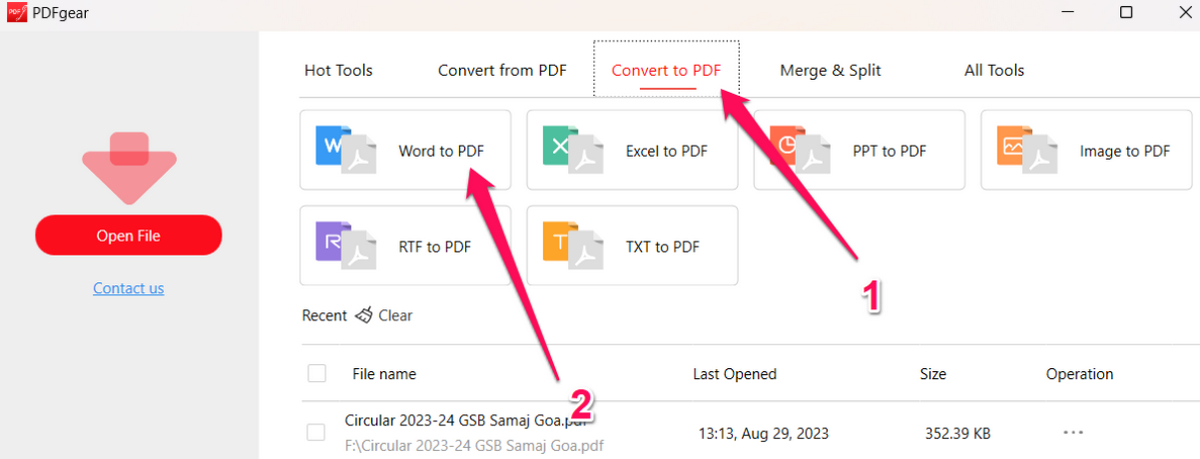Go to PDFgear PDF to Word Converter

Step 3. Add one or more PDFs with math equations, and click Convert to convert all PDFs in the queue.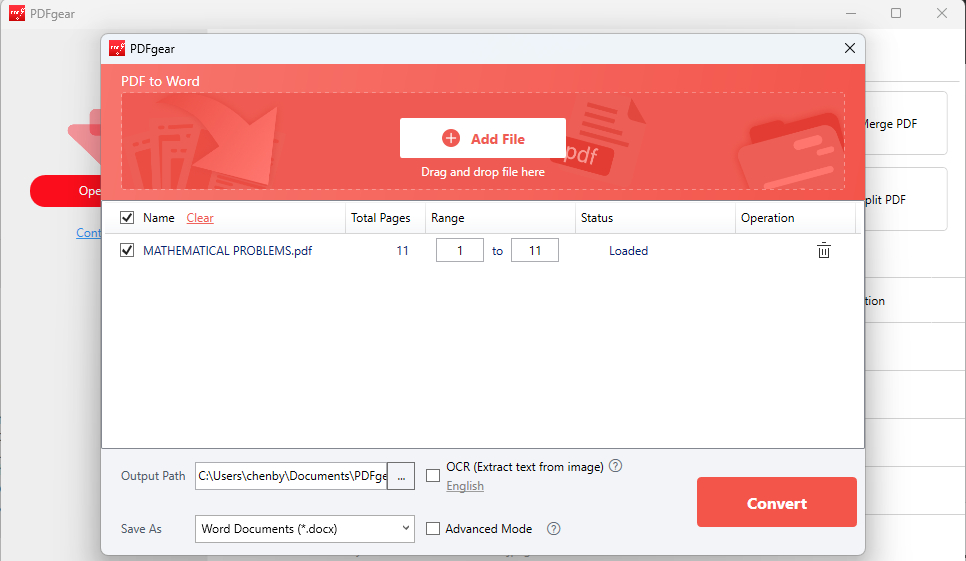Convert PDF with Equations in PDFgear

Step 4. You’ll be led to the output file folder when the conversion is done, open the Word document to check it.

Step 5. As you can see, the mathematical equations now display properly and nicely in the converted Microsoft Word document, allowing you to edit the equation and even adjust the layouts.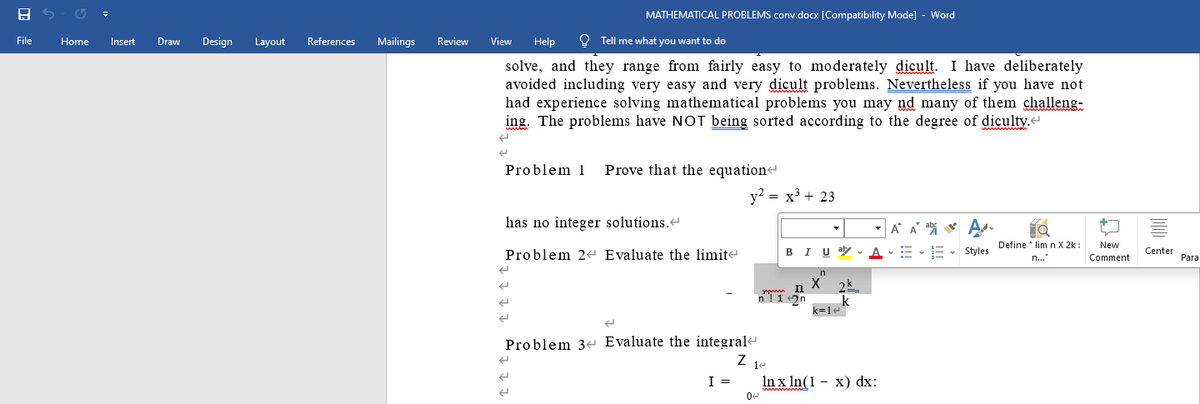Converted Word with Math Equations

## Copy and Paste the Equation Snapshot

Please note that this method is only provided as a small trick to try as it does not guarantee the result and manual adjustments to the equation text are constantly required.

However, since the process is simple and intuitive enough, I personally recommend you give it a try.

1. Open Adobe Acrobat Reader, and open the PDF you intend to work with.

2. Choose Edit from the upper menubar, go to More, and then Take A Snapshot.

3. Take a Snapshot of the equation in the PDF.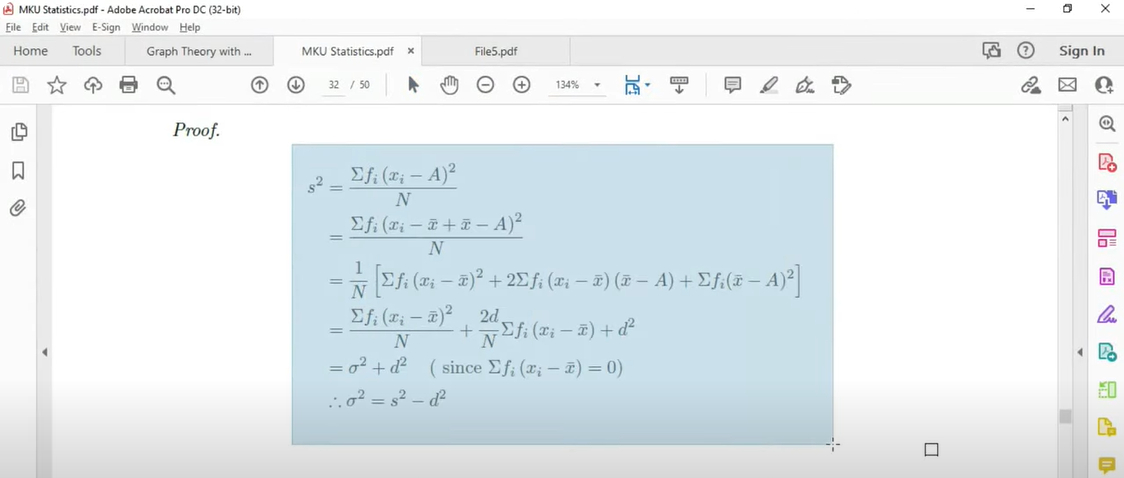Take a Snapshot for a PDF Equation

4. Open Microsoft Word and create a new Word document.

5. Right-click on the Word document, and select Paste as Math and Text.

6. If needed, make the necessary changes and alignments.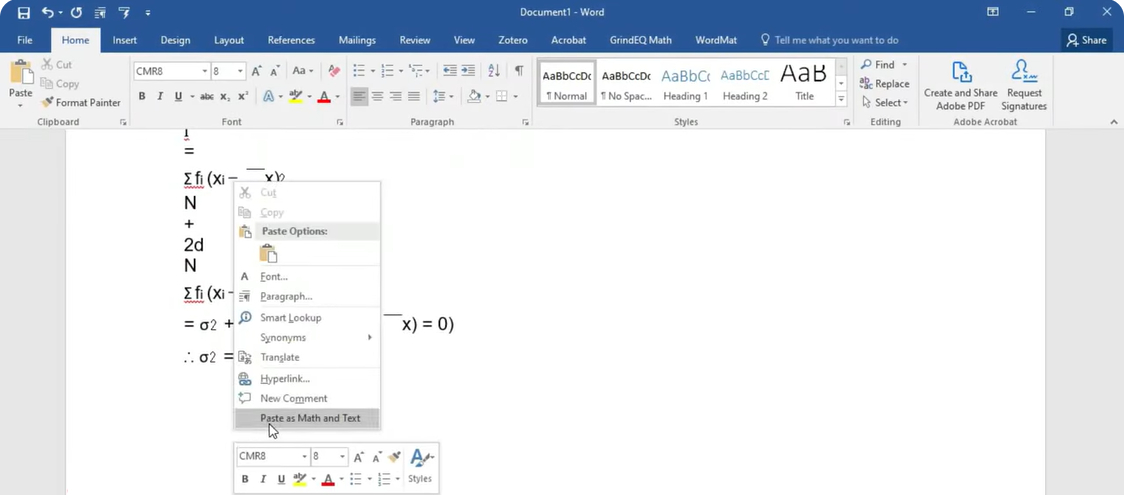Paste Equation as Math and Text

## Plain Text vs Math Equations in PDF

So, what exactly is the difference between plain text and mathematic equations in PDF documents? How come I can directly copy equations like copying PDF text to Word? Here is the answer.

PDF and Word documents handle plain text and mathematical notation differently. Plain text is typically displayed in a linear fashion while mathematical notation involves equations, symbols, and variables that require specific formatting and spacing.

As a result, copying equations between these formats can be more challenging, you can not directly copy the equation text from PDF into Microsoft Word and other document file formats.

## FAQs

### Why do equations from PDF not copy well to Word?

PDFs and Word documents are two different file formats, and the equations created within them can also differ in structure and formatting. When you try to copy an equation from a PDF to Word, the formatting may be lost or distorted, resulting in errors or inconsistent rendering.

### How do I copy an equation from a PDF to Word?

There are several methods for copying equations from a PDF to Word, but the most effective way is to use a specialized software or tool that can recognize and transfer the equation structure and formatting. Some examples of such tools include Adobe Acrobat DC, MathType, or LaTeX.

### How can I check if the equation copied from PDF to Word is accurate?

After copying an equation from a PDF to Word, it’s a good practice to double-check that the formatting and symbols have been correctly reproduced. You can compare the copied equation to the original equation in the PDF or run a spellcheck in Word to ensure there are no typographical errors.

## Conclusion

The built-in equation inserting feature in Microsoft Word is always laggy and buggy, and that’s exactly the reason that it takes so much trouble to transfer equations between documents.

Luckily, we have two useful tools to work around that, so make sure to try at least one method. Once again, these methods are free to use.